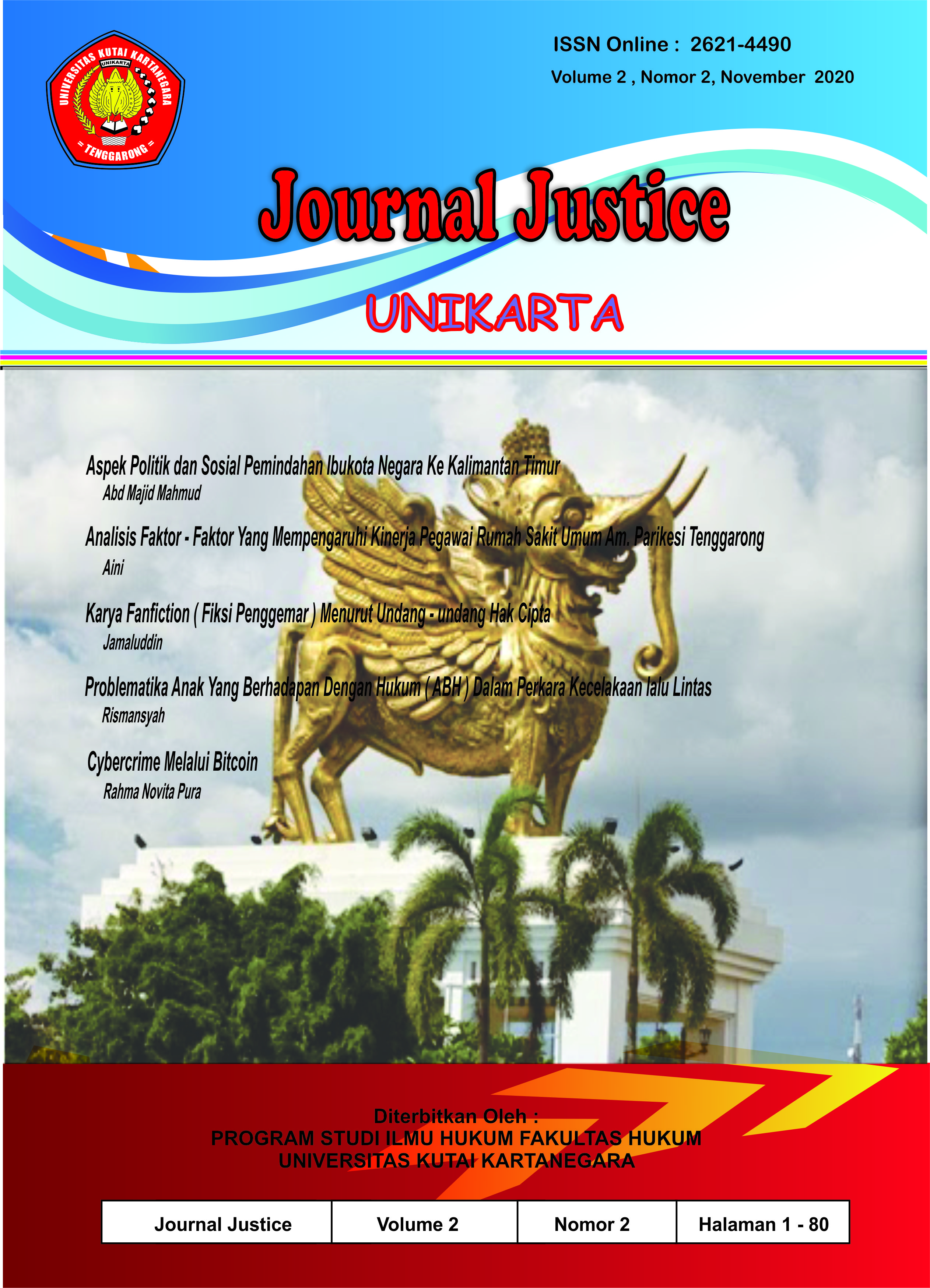# ANALISIS FAKTOR – FAKTOR YANG MEMPENGARUHI KINERJA PEGAWAI RUMAH SAKIT UMUM AM. PARIKESIT TENGGARONG

## Authors

• Aini Universitas Kutai Kartanegara

## Abstract

The results showed that it is known that the R Square number is 0.370. This means that 37% of employee performance is influenced by independent variables. Meanwhile (100% - 37% = 63%) is influenced by other factors which are not discussed in this study.

The standard error of estimate is 3.53 while the standard deviation of the variables is 4.41. Because the standard error of estimate is smaller than the standard, this regression model is better at acting as a predictor of performance than performance itself. Using the formula for the correlation coefficient measured at a significant level of 5%, it is obtained r> from the critical price of r table, namely Xi = 0.471, X2 = an X3 = 0.130> 0.207. This means that there is a positive correlation between the independent and the dependent variable.

The results of testing the hypothesis conducted using a significance level of 5% of respondents 92 - 4 - 1 = 87 obtained F count 32.281> F table 2.33. Because litas is much less than 0.05, it can be said that the factors of motivation, discipline and Lan influence the performance of the hospital employees. AM Parikesit Tenggarong.

Thus, from the results of the above calculations, the first and second hypothesis formulations can be accepted, where the hypothesis that has a dominant influence on employee performance is the second discipline where it is part of the demands of society in obtaining quality health service quality. However, motivation and education cannot be ignored because they can affect the level of professionalism and performance.

Keywords: Factors, Performance, Am. Parikesi